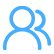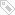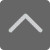TOP

深圳九年级上册数学用配方法求解一元二次方程练习题及答案

2018-12-29 10:18:52 　来源：网络

只含有一个未知数(一元)，并且未知数项的最高次数是2(二次)的整式方程叫做一元二次方程。一般形式为：ax²+bx+c=0(a≠0)，为帮助大家更深入的学习了解，小编给大家整理了深圳九年级上册数学用配方法求解一元二次方程练习题及答案，欢迎大家阅读参考。深圳九年级上册数学用配方法求解一元二次方程练习题及答案

一、选择题

1、用配方法解一元二次方程-6x-4=0，下列变形正确的是(　　)

A.=-4+36 B.=4+36 C.=-4+9 D.=4+9

答案：D

解析：解答：-6x-4=0，移项，得-6x=4，配方，得故选：D.

分析：. 本题考查了解一元一次方程，利用配方法解一元一次方程：移项.二次项系数化为1，配方的过程。

2、一元二次方程-8x-1=0配方后可变形为(　　)

A.=17 B.=15 C.=17 D.=15

答案：C

解析：解答：方程变形得：-8x=1，配方得：x-8x+16=17，即(x-4)=17，故选C.

分析：方程利用配方法求出解即可.此题考查了解一元二次方程-配方法，熟练掌握完全平方公式是解本题的关键.

3.方程=9的根是(　　)

A.x=3 B.x=-3 C.=3，=-3 D.==3

答案：C.

解析：解答：x=±3，∴，

分析：利用直接开平方法解方程.

4、代数式-4x+5的最小值是(　　)

A.-1 B.1 C.2 D.5

答案：B

解析： 解答：∵-4x+5=-4x+4-4+5=+1∵≥0，∴≥1，∴当x=2时，代数-4x+5的最小值为1.故选B.

分析：此题考查了配方法，若二次项系数为1，则常数项是一次项系数的一半的平方，若二次项系数不为1，则可先提取二次项系数，将其化为1后再计算.

5、用配方法把一元二次方程+6x+1=0，配成=q的形式，其结果是(　　)

A.=8 B.=1 C.=10 D.=4

答案：A

解析：解答：+6x =-1，+6x+9=-1+9，=8 故选A.

分析：先移项得到+6x =-1，再把方程两边加上9，然后利用完全平方公式即可得到. =8

6、二次三项式-4x+7配方的结果是(　　)

A.+7 B.+3 C.+3 D. -1

答案：B

解析： 解答：-4x+7=-4x+4+3=+3故选B.

分析：此题考查了配方法，若二次项系数为1，则常数项是一次项系数的一半的平方，若二次项系数不为1，则可先提取二次项系数，将其化为1后再计算.

7.若M=2-12x+15，N=-8x+11，则M与N的大小关系为(　　)

A.M≥N B.M>N C.M≤N D.M

答案：A

解析：解答：

M-N=(2-12x+15)-(-8x+11)，=-4x+4，=.∵≥0，∴M≥N.故选：A.

分析：利用求差法判定两式的大小，将M与N代入M-N中，去括号合并得到最简结果，根据结果的正负即可做出判断.

8、一元二次方程-2x-1=0的解是( 　)

A.==1 B.=1+，=-1-

C.=1+，=1- D.=-1+，=-1-

答案：C

解析：解答：方程-2x-1=0，变形得：-2x=1，配方得：-2x+1=2，即=2，开方得：x-1=±2，解得： =1+，=1-.故选：C.

分析：方程变形后，配方得到结果，开方即可求出值.

9、配方法解方程2?x?2=0变形正确的是(　　)

A. B. C. D.

答案：D

解析：解答：，移项得：，二次项系数化为1得：，配方得：， 故选：D.

分析：根据配方法的步骤，把方程配方即可.

10、对任意实数x，多项式-+6x-10的值是一个(　　)

A.正数 B.负数 C.非负数 D.无法确定

答案：B

解析：解答：-+6x-10=-(-6x)-10=-(-6x+9-9)-10=--1，∵-(≤0，∴--1<0，

即多项式-+6x-10的值是一个负数.

故选B.

分析：利用配方法把-+6x-10变形为--1，然后根据非负数的性质可判断-+6x-10<0.

11、用配方法解一元二次方程-4x-5=0的过程中，配方正确的是(　　)

A.=1 B.=1 C.=9 D.=9

答案：D

解析：解答：移项得：-4x=5，配方得：-4x+=5+，=9故选D.

分析：先移项，再方程两边都加上一次项系数一半的平方，即可得出答案.

12、用配方法解下列方程，其中应在方程的左右两边同时加上4的是(　　)

A.-2x=5 B.+4x=5 C.+2x=5 D.2-4x=5

答案：B

解析：解答：A.因为本方程的一次项系数是-2，所以等式两边同时加上一次项系数一半的平方1;故本选项错误;B.因为本方程的一次项系数是4，所以等式两边同时加上一次项系数一半的平方4;故本选项正确;C.因为本方程的一次项系数是2，所以等式两边同时加上一次项系数一半的平方1;故本选项错误;D.将该方程的二次项系数化为1-2x=，所以本方程的一次项系数是-2，所以等式两边同时加上一次项系数一半的平方1;故本选项错误;故选B.

分析：配方法的一般步骤：(1)把常数项移到等号的右边;(2)把二次项的系数化为1;(3)等式两边同时加上一次项系数一半的平方.

13、将一元二次方程-6x-5=0化成=b的形式，则b等于(　　)

A.4 B.-4 C.14 D.-14

答案：C

解析：解答：方程-6x-5=0，移项得：-6x=5，配方得：-6x+9=14，即=14，则b=14，故选C

分析：方程常数项移项后，两边加上一次项系数一半的平方，变形即可求出b的值.

14、已知方程-6x+8=0可配方成方程=1的形式，则-6x+8=2配成方程是(　　)

A.=-1 B.=3 C.=1 D.=1

答案：B

解析： 解答：-6x+8=0，变形得：-6x=-8，配方得：-6x+9=1，即=1，∴q=3，

-6x+8=2，配方得：-6x+9=3，=3，则-6x+8=2可配成方程是=3.

故选B

分析：已知方程配方后求出q的值，所求方程配方即可得到结果.

15、用配方法解方程-4x+3=0，下列配方正确的是(　　)

A.=1 B.=1 C.=7 D.=4

答案：A

解析：解答：方程-4x+3=0，移项得：-4x=-3，配方得：-4x+4=1，即=1，故选A

分析：方程常数项移项后，两边加上一次项系数一半的平方，变形即可求出结果.

二、填空题

16、把方程变形为的形式后，h= ，k= .

答案：3|6

解析：解答：移项，得配方，得所以，故答案是：3;6

分析：把常数项移到选号的右边;等式两边同时加上一次项系数一半的平方.

17、如果一元二次方程经过配方后，得，那么a= .

答案：-6

解析：解答：=3 即 则a= -6

分析：利用完全平方公式化简后，即可确定出a的值.

18、将变形为，则m+n= .

答案：18

解析：解答： 则m=3，n=15则m+n=3+15=18故答案为：18

分析：配方法的一般步骤：(1)把常数项移到等号的右边;(2)把二次项的系数化为1;(3)等式两边同时加上一次项系数一半的平方，把原方程变为的形式。

19、用配方法解方程，则配方后的方程是 .

答案：

解析：解答：方程变形得：配方，得：即：故答案为：

分析：方程常数项移到右边，二次项系数化为1，两边加上一次项系数一半的平方，即可求得结果。

20、如果一个三角形的三边均满足方程，则此三角形的面积是 .

答案：

解析：解答：由，得 ∴∵一个三角形的三边均满足方程 ∴此三角形是以5为边长的等边三角形，∴三角形的面积=°=故答案是：

分析：根据题意，已知方程的解是三角形的三条边的长度，根据三边关系求得三角形的形状，然后根据形状求其面积即可。

三、解答题

21、解一元二次方程

答案：，

解析：解答：由原方程，得直接开平方，得则，或解得：，

分析：先把原方程的右边转化为完全平方形式，然后直接开平方.

22、用配方法解方程：

答案：，

解析： 解答：由原方程，得，配方，得即，开方得解得：，

分析：首先把方程的二次项系数化为1，移项，然后在方程的左右两边同时加上一次项系数一半的平方，左边就是完全平方式，右边就是常数，然后利用平方根的定义即可求解。

23、我们知道：若，则x=3或x=-3.因此，小南在解方程时，采用了以下的方法：解：移项得两边都加上1，得，所以;则或所以或.小南的这种解方程方法，在数学上称之为配方法.请用配方法解方程

答案：或

解析：解答：移项得：两边都加上4，得，所以=9;则或所以或

分析：配方法的一般步骤：(1)把常数项移到等号的右边;(2)把二次项的系数化为1;(3)等式两边同时加上一次项系数一半的平方.

24、已知当x=2时，二次三项式的值等于4，那么当x为何值时，这个二次三项式的值是9?

答案：或

解析：解答：把x=2代入方程得∴m=2， 把m=2代入∴原方程的实数根为或答：当或时，这个二次三项式的值是9.

分析：把x=2代入方程求出m，把m的值代入得了关于x的方程，求出方程的解即可.

25、已知实数a，b满足，求的值.

答案：=3或-1

解析：解答：

方程变形得： 分解因式得：则=3或-1

分析：方程左边前两项利用完全平方公式变形，求出方程的解即可确定出所求式子的值。

配方法，可解全部一元二次方程，如：解方程：x^2-4x+3=0，把常数项移项得：x^2-4x=-3　　等式两边同时加1，首先要通过Δ=b^2-4ac的根的判别式来判断一元二次方程有几个根，如果同学们还想了解更多有关深圳六年级上册数学圆的面积(一)的学习问题，欢迎前往深圳学而思1对1官网进行查阅学习。请加入QQ群，试题资料免费放送！
 深圳小学交流群：254317299 一键加群 深圳初中交流群：90482695 一键加群 高一年级交流群：293049985 一键加群 高中交流群：175743089 一键加群 高二年级交流群：148082199 一键加群 2021高考交流群：644059693 一键加群标签：